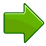c2ZWPA3kduw

# TrianglesA triangle has three sides and three anglesThe three angles always add to 180°

## Equilateral, Isosceles and Scalene

There are three special names given to triangles that tell how many sides (or angles) are equal.

There can be 3, 2 or no equal sides/angles:### Equilateral Triangle

Three equal sides
Three equal angles, always 60°### Isosceles Triangle

Two equal sides
Two equal angles### Scalene Triangle

No equal sides
No equal angles

How to remember? Alphabetically they go 3, 2, none:

• Equilateral: "equal"-lateral (lateral means side) so they have all equal sides
• Isosceles: means "equal legs", and we have two legs, right?   Also iSOSceles has two equal "Sides" joined by an "Odd" side.
• Scalene: means "uneven" or "odd", so no equal sides.

## What Type of Angle?

Triangles can also have names that tell you what type of angle is inside:### Acute Triangle

All angles are less than 90°### Right Triangle

Has a right angle (90°)### Obtuse Triangle

Has an angle more than 90°

## Combining the Names

Sometimes a triangle will have two names, for example:### Right Isosceles Triangle

Has a right angle (90°), and also two equal angles

Can you guess what the equal angles are?

## Play With It ...

Try dragging the points around and make different triangles:

geometry/images/triangle.js?mode=type

You might also like to play with the Interactive Triangle.

## Angles

The three interior angles always add to 180°

geometry/images/triangle.js?mode=angles

## Perimeter

The perimeter is the distance around the edge of the triangle: just add up the three sides:

geometry/images/triangle.js?mode=perim

## AreaThe area is half of the base times height.

• "b" is the distance along the base
• "h" is the height (measured at right angles to the base)

Area = ½ × b × h

The formula works for all triangles.

Note: a simpler way of writing the formula is bh/2

### Example: What is the area of this triangle?(Note: 12 is the height, not the length of the left-hand side)

Height = h = 12

Base = b = 20

Area = ½ × b × h = ½ × 20 × 12 = 120

The base can be any side, Just be sure the "height" is measured at right angles to the "base":

geometry/images/triangle.js?mode=area

(Note: You can also calculate the area from the lengths of all three sides using Heron's Formula.)

### Why is the Area "Half of bh"?

Imagine you "doubled" the triangle (flip it around one of the upper edges) to make a square-like shape (a parallelogram) which can be changed to a simple rectangle:THEN the whole area is bh, which is for both triangles, so just one is ½ × bh.

6702, 6708,720, 3134, 5032,627,723, 3132, 3133, 7502